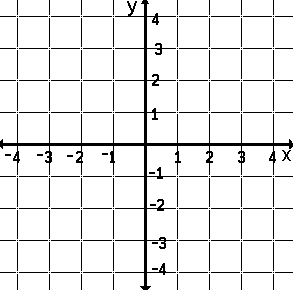A Graph is A Portrait

A Graph Is A Stylized Picture.
A graph is a stylized picture. It shows how a first set of numbers [see DOMAIN] is acted upon by a rule to create a second set of numbers [see RANGE]. Just as the Impressionists used points to paint a picture, mathematicians use points to produce their graph. Though an Impressionists' points blurr to create the picture, the mathematician's points are drawn as accurately as possible. A mathematician's blank canvas is a coordinate plane.
Remember, a graph is a stylized picture produced to display how one set of numbers is related to another. The blank canvas is the coordinate plane -- the stylized element. [See COORDINATE PLANE].
Look carefully at the blank canvas, the coordinate plane. Half way from the top to the bottom of the picture is a line, a number line [see NUMBER LINE], which acts as a base line [see X-AXIS]. Every point on the line is named by a real number [see REAL]. These are numbers which may be acted upon by the rule. If the rule acts upon a number on this line and results in a real number, a point will be placed on the canvas on, above, or below this number. If the rule does not act upon this number or if the result of the action is not a real number, no point is placed on the canvas. The exact location of the point is determined by the rule. The vertical number line [see Y-AXIS] is the scale used to place the point.Portrait of a Function -- The Graph ofMathematicians know what this graph looks like without having to see it on paper, on a calculator, or on a computer screen. Beginning math students might be required to generate the curve -- draw the graph -- create the portrait. [If you wish to see how this is done, complete this jump.] Here, we'll simply see how this is indeed a stylized picture which conveys how the domain is acted upon by the rule to produce the range. We'll consider only a few of the many points graphed.
Look at the graph of.
Notice that on the left there are no points anywhere on the canvas. This is because the results of performing the rule, square roots of the numbers, -1, -2, -3, -4, are numbers which are not real numbers --.
A student who has not yet learned about imaginary numbers would simply say, "You can't graph a point because you can't take the square root of a negative number."
Look again at the graph. On, above, or below all other points (numbers) on the horizontal axis, there is a point; the exact location of the number used. There is a point 1 unit above the 1 on the x-axis because the square root of 1 is 1. There is a point about 1.4 units above the 2 on the x-axis because the square root of 2 is about 1.4.What Is A Graph?
A graph is a means of summarizing or representing what a relation does.
If one knows the graph, one knows how the relation behaves.
Once a graph is drawn, as in statistical data collection or experimentation in a science class, it suggests what relation might exist between the two sets of numbers and permit a possible expression to be written.
Just as a picture, a graph is worth a thousand words.

This is a page from the dictionary MATH SPOKEN HERE!, published in 1995 by MATHEMATICAL CONCEPTS, inc., ISBN: 0-9623593-5-1.   You are hereby granted permission to make ONE printed copy of this page and its picture(s) for your PERSONAL and not-for-profit use.